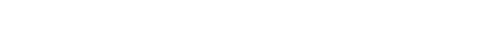glGetTexGen.3glOpenGL man pages

```

```

## Name

```  glGetTexGendv, glGetTexGenfv,	glGetTexGeniv -	return texture coordinate
generation parameters

```

## C Specification

```  void glGetTexGendv( GLenum coord,
GLenum pname,
GLdouble *params )
void glGetTexGenfv( GLenum coord,
GLenum pname,
GLfloat *params )
void glGetTexGeniv( GLenum coord,
GLenum pname,
GLint *params )

```

## Parameters

```
coord	  Specifies a texture coordinate.  Must	be GL_S, GL_T, GL_R, or	GL_Q.

pname	  Specifies the	symbolic name of the value(s) to be returned.  Must
be either GL_TEXTURE_GEN_MODE	or the name of one of the texture
generation plane equations: GL_OBJECT_PLANE or GL_EYE_PLANE.

params  Returns the requested	data.

```

## Description

```  glGetTexGen returns in params	selected parameters of a texture coordinate
generation function that was specified using glTexGen.  coord	names one of
the (s,t,r,q)	texture	coordinates, using the symbolic	constant GL_S, GL_T,
GL_R,	or GL_Q.

pname	specifies one of three symbolic	names:

GL_TEXTURE_GEN_MODE		params returns the single-valued texture
generation function, a symbolic	constant.

GL_OBJECT_PLANE		params returns the four	plane equation
coefficients that specify object linear-
coordinate generation.	Integer	values,	when
requested, are mapped directly from the
internal floating-point	representation.

GL_EYE_PLANE			params returns the four	plane equation
coefficients that specify eye linear-
coordinate generation.	Integer	values,	when
requested, are mapped directly from the
internal floating-point	representation.	 The
returned values	are those maintained in	eye
coordinates.  They are not equal to the
values specified using glTexGen, unless	the
modelview matrix was identity at the time
glTexGen was called.

```

## Notes

```  If an	error is generated, no change is made to the contents of params.

```

## Errors

```  GL_INVALID_ENUM is generated if coord	or pname is not	an accepted value.

GL_INVALID_OPERATION is generated if glGetTexGen is called between a call
to glBegin and the corresponding call	to glEnd.

```

```  glTexGen